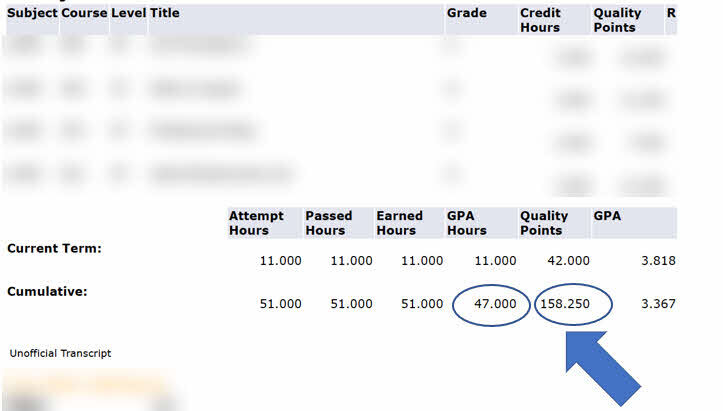### GPA Calculator

Students may use the calculator as a planning tool to help calculate their term and cumulative GPA.

##### Prior to Current Term (optional)

Obtained from Student Information System

##### Transcript Example### How To Calculate

##### Term GPA Calculation
Information Needed
• Required - The letter grade for each course in the term
• Required - The number of credit hours for each course in the term
Calculator Result

The term GPA for the current term

##### Cumulative GPA Calculation (optional)
Information Needed
• Required - Term grade data for the current term (See above)
• Required - The cumulative hours earned as noted on the Banner System - This will not include the current term hours earned
• Required - The cumulative GPA quality points earned as noted on the Banner System - This will not include the current term quality points earned
Calculator Result

The term GPA for the current term, and the student's cumulative GPA.

The following Quality Points are earned for each Hour given a certain letter grade.

• A = 4
• A- = 3.75
• B+ = 3.5
• B = 3
• B- = 2.75
• C+ = 2.5
• C = 2
• C- = 1.75
• D+ = 1.5
• D = 1
• D- = 0.75
• F = 0